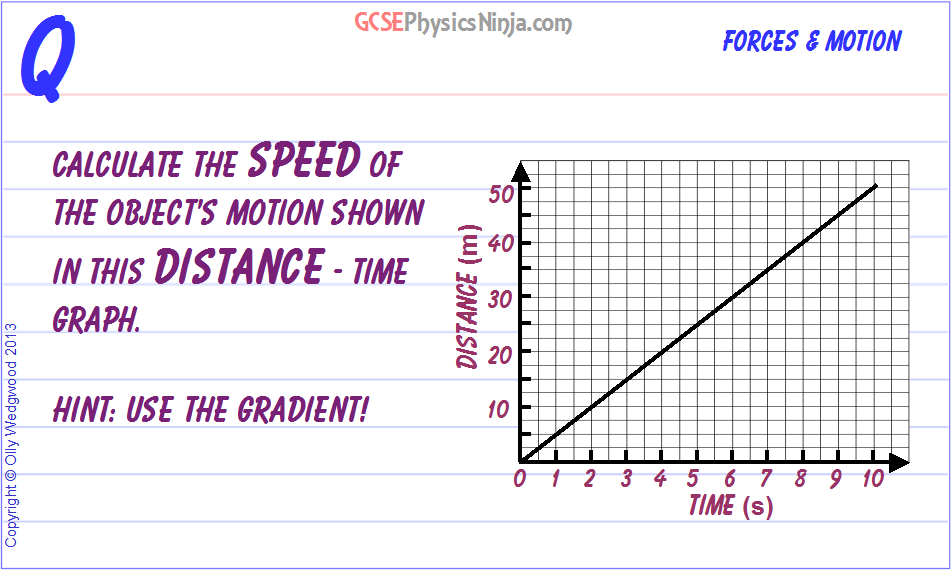# 17. Calculating speed from a distance time graph-

``` ```

## Calculating the speed from a distance-time graph

To find the speed of an object from a distance-time graph, we need to calculate the gradient (or slope) of the line.

First choose two points that lie the line - say point A is (0 , 0) and point B is (10 , 50). These are quite far apart so that will make our gradient calculation more accurate.

Now draw a right-angled triangle with two horizontal and vertical sides. Starting at point A, we move 10 seconds along the base of the triangle, so the 'run' is +10 secs.

Now move up the vertical side of the triangle a distance of 50 metres. This is our 'rise' = +50m

Use the formula: Gradient = Rise/Run and sub-in the numbers

= 50/10

= 5

The speed shown in this distance time graph is therefore 5 m/s.

(Note that you could also have used the formula: Average Speed = Distance travelled / Time taken
This would have given us the same result.)

GCSE Physics Keywords: Gradient, Rise, Run, Distance, Time, Speed

Course overview